Anzeige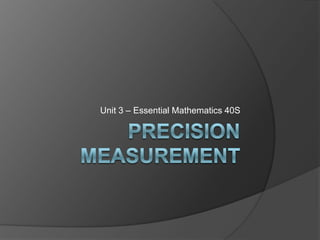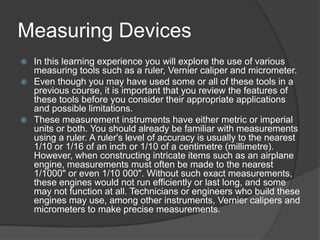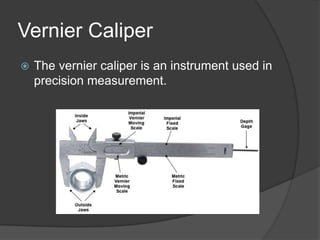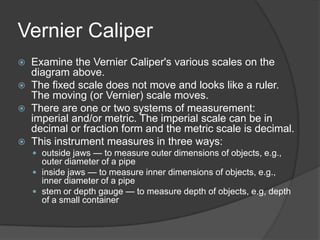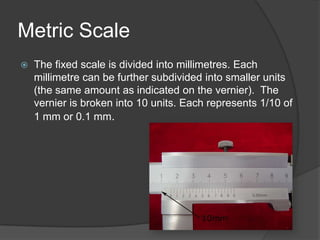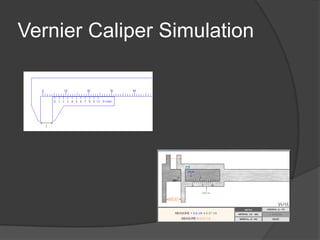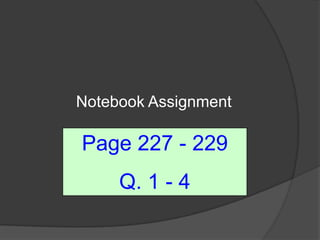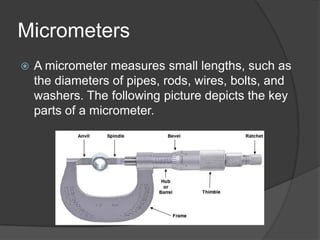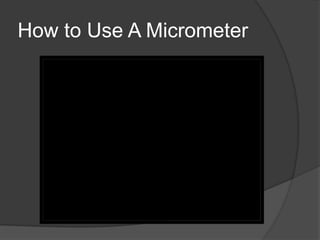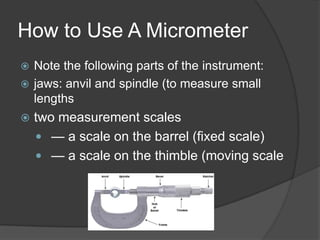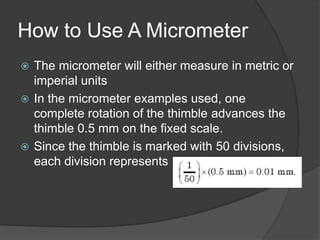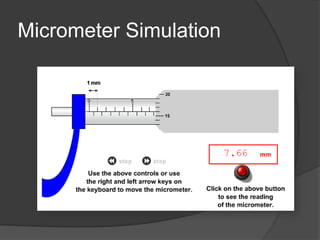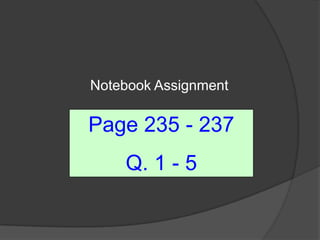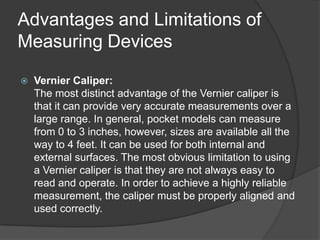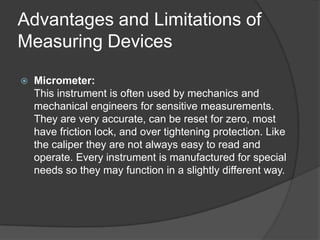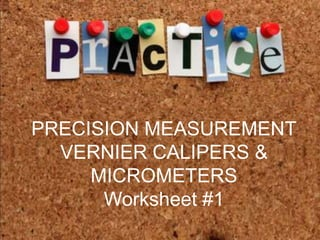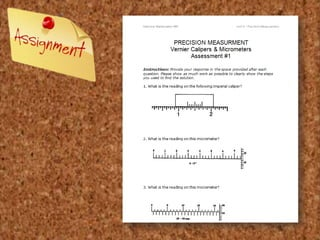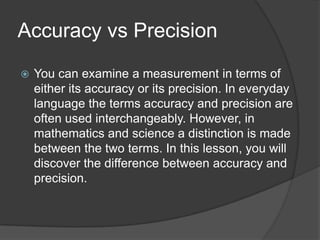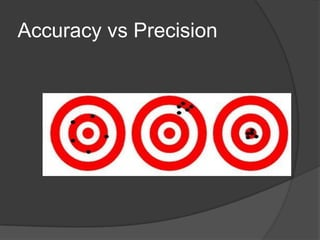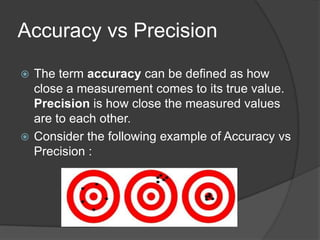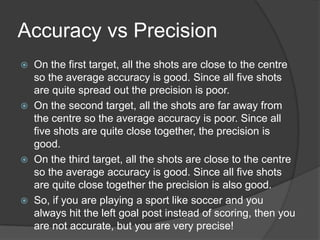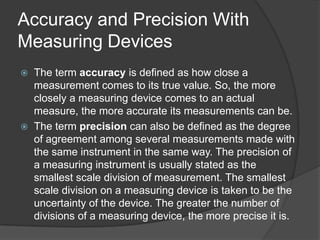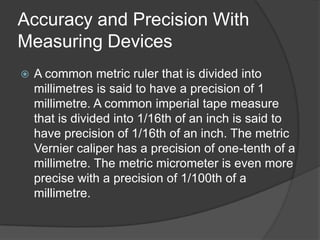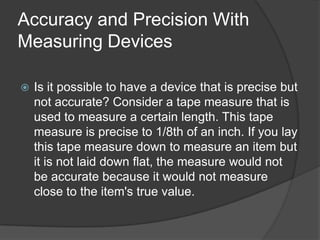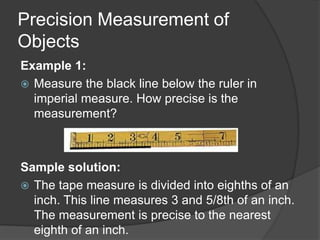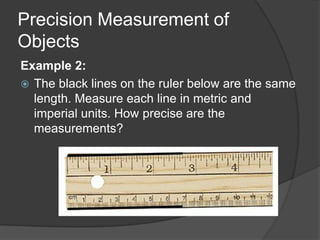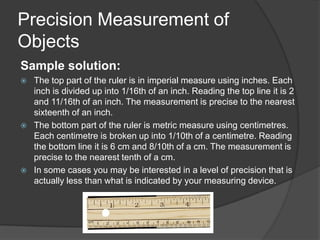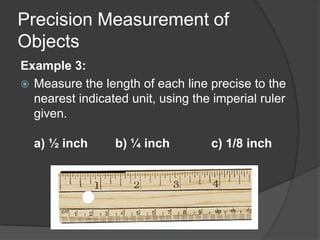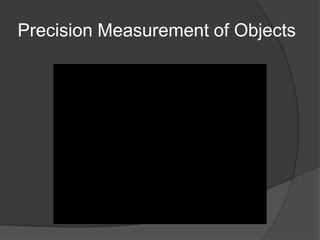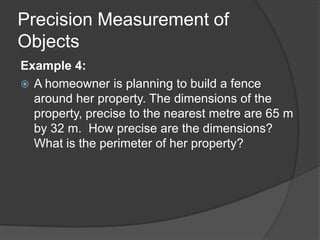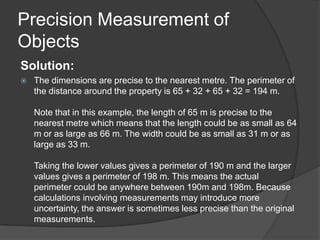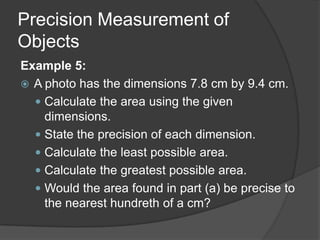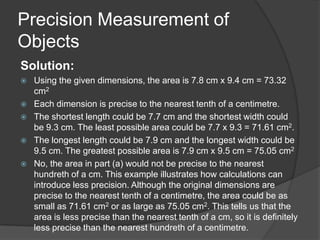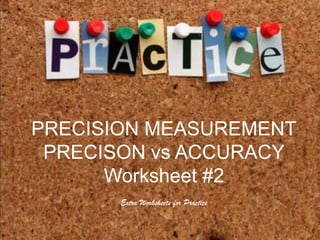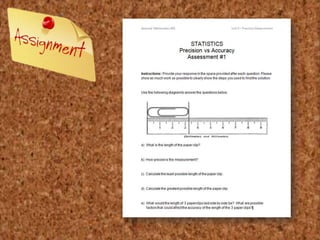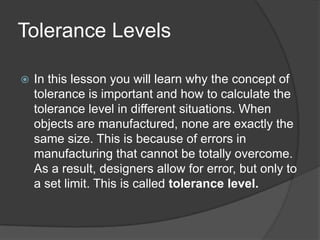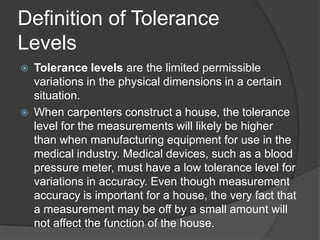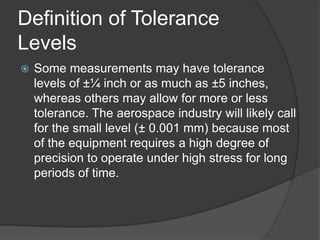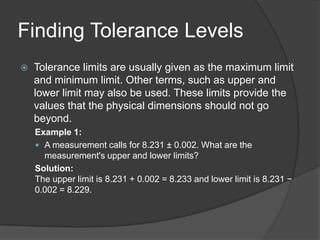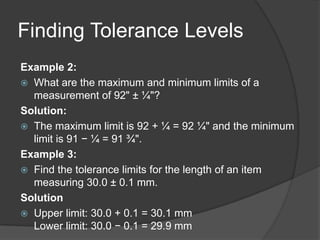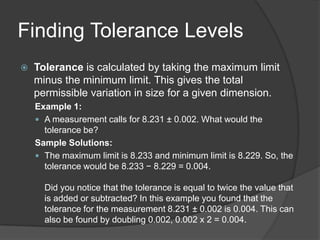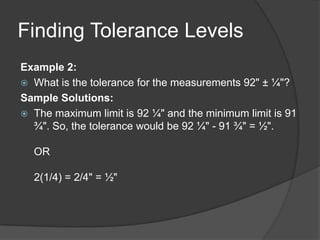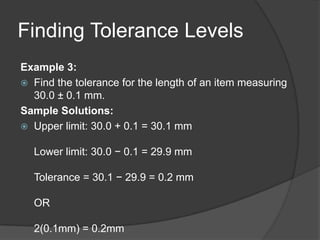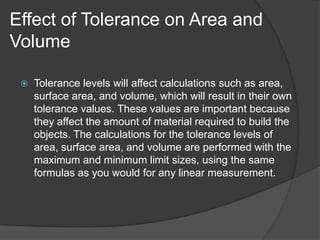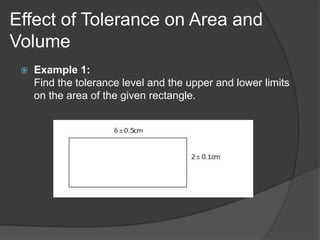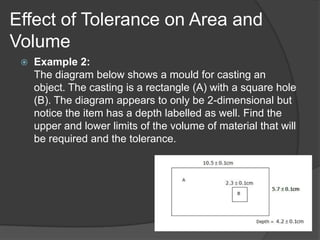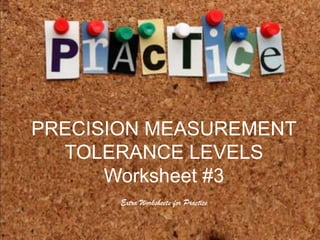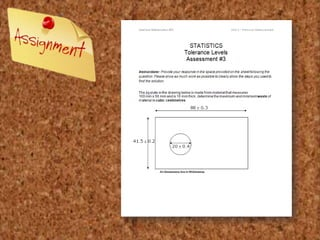1 von 49
Anzeige

### Unit 3 Precision Measurement

1. Unit 3 – Essential Mathematics 40S
2. Measuring Devices  In this learning experience you will explore the use of various measuring tools such as a ruler, Vernier caliper and micrometer.  Even though you may have used some or all of these tools in a previous course, it is important that you review the features of these tools before you consider their appropriate applications and possible limitations.  These measurement instruments have either metric or imperial units or both. You should already be familiar with measurements using a ruler. A ruler's level of accuracy is usually to the nearest 1/10 or 1/16 of an inch or 1/10 of a centimetre (millimetre). However, when constructing intricate items such as an airplane engine, measurements must often be made to the nearest 1/1000" or even 1/10 000". Without such exact measurements, these engines would not run efficiently or last long, and some may not function at all. Technicians or engineers who build these engines may use, among other instruments, Vernier calipers and micrometers to make precise measurements.
3. Vernier Caliper  The vernier caliper is an instrument used in precision measurement.
4. Vernier Caliper  Examine the Vernier Caliper's various scales on the diagram above.  The fixed scale does not move and looks like a ruler. The moving (or Vernier) scale moves.  There are one or two systems of measurement: imperial and/or metric. The imperial scale can be in decimal or fraction form and the metric scale is decimal.  This instrument measures in three ways:  outside jaws — to measure outer dimensions of objects, e.g., outer diameter of a pipe  inside jaws — to measure inner dimensions of objects, e.g., inner diameter of a pipe  stem or depth gauge — to measure depth of objects, e.g, depth of a small container
5. Vernier Caliper  Examine the Vernier Caliper's various scales on the diagram above.  The fixed scale does not move and looks like a ruler. The moving (or Vernier) scale moves.  There are one or two systems of measurement: imperial and/or metric. The imperial scale can be in decimal or fraction form and the metric scale is decimal.  This instrument measures in three ways:  outside jaws — to measure outer dimensions of objects, e.g., outer diameter of a pipe  inside jaws — to measure inner dimensions of objects, e.g., inner diameter of a pipe  stem or depth gauge — to measure depth of objects, e.g, depth of a small container
6. Metric Scale  The fixed scale is divided into millimetres. Each millimetre can be further subdivided into smaller units (the same amount as indicated on the vernier). The vernier is broken into 10 units. Each represents 1/10 of 1 mm or 0.1 mm.
7. Vernier Caliper Simulation
8. Notebook Assignment Page 227 - 229 Q. 1 - 4
9. Micrometers  A micrometer measures small lengths, such as the diameters of pipes, rods, wires, bolts, and washers. The following picture depicts the key parts of a micrometer.
10. How to Use A Micrometer
11. How to Use A Micrometer  Note the following parts of the instrument:  jaws: anvil and spindle (to measure small lengths  two measurement scales  — a scale on the barrel (fixed scale)  — a scale on the thimble (moving scale
12. How to Use A Micrometer  The micrometer will either measure in metric or imperial units  In the micrometer examples used, one complete rotation of the thimble advances the thimble 0.5 mm on the fixed scale.  Since the thimble is marked with 50 divisions, each division represents
13. Micrometer Simulation
14. Notebook Assignment Page 235 - 237 Q. 1 - 5
15. Advantages and Limitations of Measuring Devices  Vernier Caliper: The most distinct advantage of the Vernier caliper is that it can provide very accurate measurements over a large range. In general, pocket models can measure from 0 to 3 inches, however, sizes are available all the way to 4 feet. It can be used for both internal and external surfaces. The most obvious limitation to using a Vernier caliper is that they are not always easy to read and operate. In order to achieve a highly reliable measurement, the caliper must be properly aligned and used correctly.
16. Advantages and Limitations of Measuring Devices  Micrometer: This instrument is often used by mechanics and mechanical engineers for sensitive measurements. They are very accurate, can be reset for zero, most have friction lock, and over tightening protection. Like the caliper they are not always easy to read and operate. Every instrument is manufactured for special needs so they may function in a slightly different way.
17. Practice  Lorem ipsum dolor sit amet, consectetuer adipiscing elit. Vivamus et magna. Fusce sed sem sed magna suscipit egestas.  PRECISION MEASUREMENT Lorem ipsum dolor sit amet, consectetuer adipiscing elit. Vivamus & VERNIER CALIPERS et magna. Fusce sed sem sed magna MICROMETERS suscipit egestas. Worksheet #1
18. Practice  Lorem ipsum dolor sit amet, consectetuer adipiscing elit. Vivamus et magna. Fusce sed sem sed magna suscipit egestas.  Lorem ipsum dolor sit amet, consectetuer adipiscing elit. Vivamus et magna. Fusce sed sem sed magna suscipit egestas.
19. Accuracy vs Precision  You can examine a measurement in terms of either its accuracy or its precision. In everyday language the terms accuracy and precision are often used interchangeably. However, in mathematics and science a distinction is made between the two terms. In this lesson, you will discover the difference between accuracy and precision.
20. Accuracy vs Precision
21. Accuracy vs Precision  The term accuracy can be defined as how close a measurement comes to its true value. Precision is how close the measured values are to each other.  Consider the following example of Accuracy vs Precision :
22. Accuracy vs Precision  On the first target, all the shots are close to the centre so the average accuracy is good. Since all five shots are quite spread out the precision is poor.  On the second target, all the shots are far away from the centre so the average accuracy is poor. Since all five shots are quite close together, the precision is good.  On the third target, all the shots are close to the centre so the average accuracy is good. Since all five shots are quite close together the precision is also good.  So, if you are playing a sport like soccer and you always hit the left goal post instead of scoring, then you are not accurate, but you are very precise!
23. Accuracy and Precision With Measuring Devices  The term accuracy is defined as how close a measurement comes to its true value. So, the more closely a measuring device comes to an actual measure, the more accurate its measurements can be.  The term precision can also be defined as the degree of agreement among several measurements made with the same instrument in the same way. The precision of a measuring instrument is usually stated as the smallest scale division of measurement. The smallest scale division on a measuring device is taken to be the uncertainty of the device. The greater the number of divisions of a measuring device, the more precise it is.
24. Accuracy and Precision With Measuring Devices  A common metric ruler that is divided into millimetres is said to have a precision of 1 millimetre. A common imperial tape measure that is divided into 1/16th of an inch is said to have precision of 1/16th of an inch. The metric Vernier caliper has a precision of one-tenth of a millimetre. The metric micrometer is even more precise with a precision of 1/100th of a millimetre.
25. Accuracy and Precision With Measuring Devices  Is it possible to have a device that is precise but not accurate? Consider a tape measure that is used to measure a certain length. This tape measure is precise to 1/8th of an inch. If you lay this tape measure down to measure an item but it is not laid down flat, the measure would not be accurate because it would not measure close to the item's true value.
26. Precision Measurement of Objects Example 1:  Measure the black line below the ruler in imperial measure. How precise is the measurement? Sample solution:  The tape measure is divided into eighths of an inch. This line measures 3 and 5/8th of an inch. The measurement is precise to the nearest eighth of an inch.
27. Precision Measurement of Objects Example 2:  The black lines on the ruler below are the same length. Measure each line in metric and imperial units. How precise are the measurements?
28. Precision Measurement of Objects Sample solution:  The top part of the ruler is in imperial measure using inches. Each inch is divided up into 1/16th of an inch. Reading the top line it is 2 and 11/16th of an inch. The measurement is precise to the nearest sixteenth of an inch.  The bottom part of the ruler is metric measure using centimetres. Each centimetre is broken up into 1/10th of a centimetre. Reading the bottom line it is 6 cm and 8/10th of a cm. The measurement is precise to the nearest tenth of a cm.  In some cases you may be interested in a level of precision that is actually less than what is indicated by your measuring device.
29. Precision Measurement of Objects Example 3:  Measure the length of each line precise to the nearest indicated unit, using the imperial ruler given. a) ½ inch b) ¼ inch c) 1/8 inch
30. Precision Measurement of Objects
31. Precision Measurement of Objects Example 4:  A homeowner is planning to build a fence around her property. The dimensions of the property, precise to the nearest metre are 65 m by 32 m. How precise are the dimensions? What is the perimeter of her property?
32. Precision Measurement of Objects Solution:  The dimensions are precise to the nearest metre. The perimeter of the distance around the property is 65 + 32 + 65 + 32 = 194 m. Note that in this example, the length of 65 m is precise to the nearest metre which means that the length could be as small as 64 m or as large as 66 m. The width could be as small as 31 m or as large as 33 m. Taking the lower values gives a perimeter of 190 m and the larger values gives a perimeter of 198 m. This means the actual perimeter could be anywhere between 190m and 198m. Because calculations involving measurements may introduce more uncertainty, the answer is sometimes less precise than the original measurements.
33. Precision Measurement of Objects Example 5:  A photo has the dimensions 7.8 cm by 9.4 cm.  Calculate the area using the given dimensions.  State the precision of each dimension.  Calculate the least possible area.  Calculate the greatest possible area.  Would the area found in part (a) be precise to the nearest hundreth of a cm?
34. Precision Measurement of Objects Solution:  Using the given dimensions, the area is 7.8 cm x 9.4 cm = 73.32 cm2  Each dimension is precise to the nearest tenth of a centimetre.  The shortest length could be 7.7 cm and the shortest width could be 9.3 cm. The least possible area could be 7.7 x 9.3 = 71.61 cm2.  The longest length could be 7.9 cm and the longest width could be 9.5 cm. The greatest possible area is 7.9 cm x 9.5 cm = 75.05 cm2  No, the area in part (a) would not be precise to the nearest hundreth of a cm. This example illustrates how calculations can introduce less precision. Although the original dimensions are precise to the nearest tenth of a centimetre, the area could be as small as 71.61 cm2 or as large as 75.05 cm2. This tells us that the area is less precise than the nearest tenth of a cm, so it is definitely less precise than the nearest hundreth of a centimetre.
35. Practice  Lorem ipsum dolor sit amet, consectetuer adipiscing elit. Vivamus et magna. Fusce sed sem sed magna suscipit egestas.  PRECISION MEASUREMENT Lorem ipsum dolor sit amet, consectetuer adipiscing ACCURACY PRECISON vs elit. Vivamus et magna. Fusce sed sem sed magna Worksheet #2 suscipit egestas. Extra Worksheets for Practice
36. Practice  Lorem ipsum dolor sit amet, consectetuer adipiscing elit. Vivamus et magna. Fusce sed sem sed magna suscipit egestas.  Lorem ipsum dolor sit amet, consectetuer adipiscing elit. Vivamus et magna. Fusce sed sem sed magna suscipit egestas.
37. Tolerance Levels  In this lesson you will learn why the concept of tolerance is important and how to calculate the tolerance level in different situations. When objects are manufactured, none are exactly the same size. This is because of errors in manufacturing that cannot be totally overcome. As a result, designers allow for error, but only to a set limit. This is called tolerance level.
38. Definition of Tolerance Levels  Tolerance levels are the limited permissible variations in the physical dimensions in a certain situation.  When carpenters construct a house, the tolerance level for the measurements will likely be higher than when manufacturing equipment for use in the medical industry. Medical devices, such as a blood pressure meter, must have a low tolerance level for variations in accuracy. Even though measurement accuracy is important for a house, the very fact that a measurement may be off by a small amount will not affect the function of the house.
39. Definition of Tolerance Levels  Some measurements may have tolerance levels of ±¼ inch or as much as ±5 inches, whereas others may allow for more or less tolerance. The aerospace industry will likely call for the small level (± 0.001 mm) because most of the equipment requires a high degree of precision to operate under high stress for long periods of time.
40. Finding Tolerance Levels  Tolerance limits are usually given as the maximum limit and minimum limit. Other terms, such as upper and lower limit may also be used. These limits provide the values that the physical dimensions should not go beyond. Example 1:  A measurement calls for 8.231 ± 0.002. What are the measurement's upper and lower limits? Solution: The upper limit is 8.231 + 0.002 = 8.233 and lower limit is 8.231 − 0.002 = 8.229.
41. Finding Tolerance Levels Example 2:  What are the maximum and minimum limits of a measurement of 92" ± ¼"? Solution:  The maximum limit is 92 + ¼ = 92 ¼" and the minimum limit is 91 − ¼ = 91 ¾". Example 3:  Find the tolerance limits for the length of an item measuring 30.0 ± 0.1 mm. Solution  Upper limit: 30.0 + 0.1 = 30.1 mm Lower limit: 30.0 − 0.1 = 29.9 mm
42. Finding Tolerance Levels  Tolerance is calculated by taking the maximum limit minus the minimum limit. This gives the total permissible variation in size for a given dimension. Example 1:  A measurement calls for 8.231 ± 0.002. What would the tolerance be? Sample Solutions:  The maximum limit is 8.233 and minimum limit is 8.229. So, the tolerance would be 8.233 − 8.229 = 0.004. Did you notice that the tolerance is equal to twice the value that is added or subtracted? In this example you found that the tolerance for the measurement 8.231 ± 0.002 is 0.004. This can also be found by doubling 0.002, 0.002 x 2 = 0.004.
43. Finding Tolerance Levels Example 2:  What is the tolerance for the measurements 92" ± ¼"? Sample Solutions:  The maximum limit is 92 ¼" and the minimum limit is 91 ¾". So, the tolerance would be 92 ¼" - 91 ¾" = ½". OR 2(1/4) = 2/4" = ½"
44. Finding Tolerance Levels Example 3:  Find the tolerance for the length of an item measuring 30.0 ± 0.1 mm. Sample Solutions:  Upper limit: 30.0 + 0.1 = 30.1 mm Lower limit: 30.0 − 0.1 = 29.9 mm Tolerance = 30.1 − 29.9 = 0.2 mm OR 2(0.1mm) = 0.2mm
45. Effect of Tolerance on Area and Volume  Tolerance levels will affect calculations such as area, surface area, and volume, which will result in their own tolerance values. These values are important because they affect the amount of material required to build the objects. The calculations for the tolerance levels of area, surface area, and volume are performed with the maximum and minimum limit sizes, using the same formulas as you would for any linear measurement.
46. Effect of Tolerance on Area and Volume  Example 1: Find the tolerance level and the upper and lower limits on the area of the given rectangle.
47. Effect of Tolerance on Area and Volume  Example 2: The diagram below shows a mould for casting an object. The casting is a rectangle (A) with a square hole (B). The diagram appears to only be 2-dimensional but notice the item has a depth labelled as well. Find the upper and lower limits of the volume of material that will be required and the tolerance.
48. Practice  Lorem ipsum dolor sit amet, consectetuer adipiscing elit. Vivamus et magna. Fusce sed sem sed magna suscipit egestas.  PRECISION MEASUREMENT Lorem ipsum dolor sit amet, consectetuer adipiscing elit. Vivamus et TOLERANCE LEVELS magna. Fusce sed sem sed magna Worksheet #3 suscipit egestas. Extra Worksheets for Practice
49. Practice  Lorem ipsum dolor sit amet, consectetuer adipiscing elit. Vivamus et magna. Fusce sed sem sed magna suscipit egestas.  Lorem ipsum dolor sit amet, consectetuer adipiscing elit. Vivamus et magna. Fusce sed sem sed magna suscipit egestas.
Anzeige